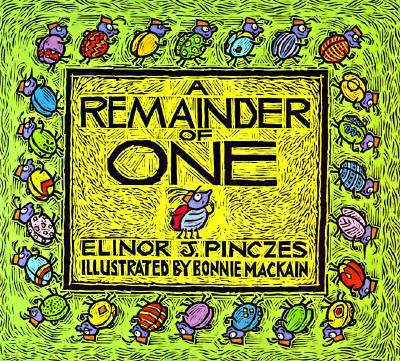Recommended Books

Filter books by:

 Strand NumberAlgebraMeasurementSpaceStatistics and ProbabilityProfessional ReadingProblem Solving Year Year 5Year 6Year 7Year 2Year 3Year 8Year 4Foundation YearYear 1Year 9Year 10Maths AMaths B Numeracy Strategy Multiplication and Division TriangleAct it OutPart-Part-WholeLook for a patternAdditive to Multiplicative ThinkingBenchmarkingSee the Parts to match the wholeNumber LineTables and GraphsDraw a diagramVisualisationFind the PatternDraw what you can't seeMental StrategiesVariousA Remainder of One

Australian Curriculum:  Description

Number and Algebra : Number and Place Value – Solve problems involving division by a one digit number, including those that result in a remainder (ACMNA101); Investigate index notation and represent whole numbers as products of powers of prime numbers (ACMNA149)

Teaching ideas
* Have students mathematically represent the ideas in the book. i.e. concrete with actual bugs, representational with tally marks and abstract would be the mathematical numbers and symbols (25 ÷ 2 = 12 r1; 25 ÷ 3 = 8 r1; 25 ÷ 4 = 6 r1; 25 ÷ 5 = 5). Year 7s could use this as an introduction to prime numbers and non-prime numbers. What are the factors of 24 and 25.Divide & Ride

Australian Curriculum:  Description

Solve problems involving division by a one digit number, including those that result in a remainder (ACMNA101)

Teaching ideas
"Student investigate other ""Total Amounts"". How many people can go on the ride? What is the best number of people to go to the fair so that there is nobody sitting alone?"